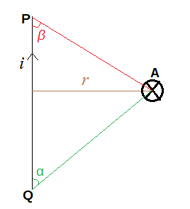## FANDOM

182 Pages

 The Biot-Savart Law relates magnetic fields to the electric current that produces it. It is applicable for small current elements.

Consider a small current carrying element ($\overline{dl}$) of the conductor XY carrying current I and P be the observation point at a distance r and making an angle $\theta$ with it as shown in diagram. Then according to Biot-Savart Law, magnetic field $\overline{dB}$ is:-

1. directly proportional to the current
$\overline{dB} \propto I$ ... (1)
1. directlly proportional to length
$\overline{dB} \propto \overline{dl}$ ...(2)
1. directly proportional to $\sin{\theta}$ of the angle between them
$\overline{dB} \propto \sin{\theta}$ ...(3)
1. inversely proportional to the square of the distance
$\overline{dB} \propto \frac{1}{r^2}$ ...(4)

Combining equations 1, 2, 3 and 4:

$\overline{dB} \propto \frac{Idl \sin{\theta} }{r^2}$
or $\overline{dB} = k\frac{Idl \sin{\theta} }{r^2}$

where 'k' is a constant whose value depends on the nature of medium and system of units.

In SI $k = \frac{\mu_o}{4\pi} = 10^{-7}$ TA-1m. $\mu_o$ is called absolute permittivity of free space.

## Magnetic field from some important cases

### CASE 1: Field of a straight infinite current wire

The magnetic field due to a current carrying straight wire of infinite length at a distance r from the wire is $B = \frac{\mu_0 i}{2 \pi r}$

The magnetic lines of force for a long straight wire are concentric circles centred at the wire and lying in the plane perpendicular to the wire. If the wire is gripped in the right hand with the thumb in direction of current the curling fingers give the direction of lines of force in circles.

#### DERIVATION

Consider Derivation for CASE 2

### CASE 2: Field of a straight current carrying wire of finite lengthConsider a straight wire PQ carrying a current i as shown. The magnetic induction at a distance r from the wire is given by:
$B = \frac{ \mu_0 i}{ 2 \pi r} \bigg( \frac{ \cos{\alpha} + \cos{\beta} }{2} \bigg)$

where α, β are the angles between the wire and the lines joining the end points of wire to the point A where the field is to be calculated.

#### DERIVATION

Consider a conductor XY carrying current I in the direction from X to Y and P be the observation point at a distance 'a' from the straight conductor.

To find magnetic field consider a small current element $I \overrightarrow{dl}$ at a distance l making an angle θ as shown.

According to Biot Savart Law, magnetic field at point P is:

$dB = \frac{ \mu_0}{4 \pi} \frac{Idl \sin{\theta} }{r^2}$

Let Φ1 and Φ2 be the angles wich point P makes with X and Y and Φ be the angle that P makes with dl.

Now, θ + Φ = 90o

So, sinθ = sin(90o - φ) = cosφ ...(1)

Also $\frac{l}{a} = \tan{\phi}$

or$l = a \tan{\phi}$

$dl = a \sec^2{\phi} d \phi$

### CASE 3: Field due to a circular current loop

Consider a circular loop of radius r carrying current i and having N turns.

(a) Field at axis:
$B = \frac{ \mu_0 i r^2 N }{2(x^2 + r^2)^{ \frac{3}{2} } }$
(b) Field at centre:
$B = \frac{\mu_0 i r^2 N}{2r}$

### CASE 4: Field at the centre of a current arc

The magnetic field at the centre of a wire PQ of length l bent in a shape of a arc of radius r is:

$B = \frac{\mu_0 i}{2r} \bigg( \frac{ \alpha }{2 \pi} \bigg) = \frac{\mu_0i}{4 \pi r^2} l$

### CASE 5: Field inside a long solenoid

Consider a solenoid of length l and radius of cross section r (r<<l) having N turns. If I is the current passing through the solenoid, the magnetic induction inside the solenoid is given as:

$B = \frac{\mu_0 I N}{l}$
or $B = \mu_0In$, where n is the number of turns per unit length.